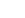# JavaScript Operators

Using operators we can combine several variables. For example basic arithmetic actions are operators. With the `plus (+)` operator two numbers are combined and the result is the sum of both numbers. We differentiate between different types of operators depending on the variable-types that are involved.

## Arithmetic Operators

This type of operators operates with numeric variables. You should make sure that real numeric variables are used because otherwise you could get an error message (`"5"` is a string, `5` is a number). The following table shows all basic arithmetic operators with some examples.

Operator Description Example Result (value of x)
`+` Addition x = 5 + 6 11
`-` Subtraction x = 6 - 5 1
`*` Multiplication x = 5 * 6 30
`/` Division x = 6 / 3 2
`%` Modulus (division remainder) x = 7 % 3 1
`++` Increment a = 7
x = a++
8
`--` Decrement a = 7
x = a--
6

If you want to increase a variable by a certain value you can use the following base construct.

The value of `number` + 1 is assigned to `number`. It often happens in JavaScript that something has to be increased or decreased by 1. That's why JavaScript offers two abbreviations: `++` and `--`. Note that you can use `variable++` and `++variable`. Both options will have the same results.

If you want to increase or decrease a variable by another number than 1 you can use another abbreviation. This abbreviation works for all basic arithmetic operations and the modulo operator.

Operator Signification Long form Abbreviation
`+=` Addition x = x + y x += b
`-=` Subtraction x = x - y x -= b
`*=` Multiplication x = x * y x *= b
`/=` Division x = x / y x /= b
`%=` Modulo x = x % y x %= b

## Boolean operators

With Boolean operators you can link Boolean values. In the following section we'll explain every single operator.

### && (and)

Only if both involved variables have the value `true` the output of the operation is `true`. All other cases will output `false`.

``````var yes = true;
var no = false;
var bool1 = yes && no; //output is false
var bool2 = yes && yes; //output is true
``````

### || (or)

If one of the involved variables is `true` the output of the operation is `true`. Only if all variables are `false` the output is `false`.

``````var yes = true;
var no = false;
var second_no = false;
var bool1 = yes || no || second_no; //output is true
var bool2 = no || second_no; //output is false
``````

### ! (negation)

The negation-operator transforms `true` into `false` and `false` into `true`.

``````var yes = true;
var no = false;
var bool1 = !yes; //output is false
var bool2 = !no; //output is true
``````

## Comparison Operators

Comparison operators are usually used for numeric values. You can also use these operators for strings.

Operator Description Examplle Result (value of x)
`==` Equal x = (5 == 6)
false
`!=` Not equal x = (5 != 6)
1
`>` Greater than x = (5 > 6) false
`<` Less than x = (5 < 6) true
`>=` Greater than or equal x = (5 >= 6) false
`<=` Less than or equal x = (5 <= 6) true

A common source of error is mixing up the assignment `=` and the equal-operator `==`. If you use a simple equal sign `=` in a comparison you will get an error message.

## String Operators

We can combine multiple variables using the `+`-operator.

``````var start = "Tool";
var end = "infy";
var full = start + end; //output is Toolinfy
``````

You can't use other arithmetic operators for strings but there are some other things you can do with them:

• `string.length` returns the number of characters that the string has.
• `string.charAt(x)` returns the character that is on position `x` in the string. The counting starts at 0. For example the fifth characters is accessed with `string.charAt(4)`.
• `string.substring(start, end)` returns a substring beginning from `start` and ending at `end`. The counting also starts at 0.

### Example

``````var test = "Toolinfy";
var x = test.charAt(0); //x == "T"
var y = test.substring(1, test.length); // b == "oolinfy";
``````